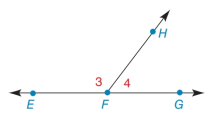Chapter 2.2, Problem 25E### Elementary Geometry for College St...

6th Edition
Daniel C. Alexander + 1 other
ISBN: 9781285195698

#### Solutions

Chapter
Section### Elementary Geometry for College St...

6th Edition
Daniel C. Alexander + 1 other
ISBN: 9781285195698
Textbook Problem
1 views

# In Exercises 23 to 34, give the indirect proof for each problem or statement. Given: m ∠ 3 > m ∠ 4 Prove: F H → is not ⊥ to E G ↔To determine

To prove:

The given statement.

Explanation

Given:

The given statement is,

m3>m4

Figure (1)

Property:

Perpendicular lines intersect each other at a angle of 90°.

Approach:

Assume that FH is to EG.

From figure (1), m3=90 and m4=90.

m3=m4

The above result contradicts the statement, m3>m4

### Still sussing out bartleby?

Check out a sample textbook solution.

See a sample solution

#### The Solution to Your Study Problems

Bartleby provides explanations to thousands of textbook problems written by our experts, many with advanced degrees!

Get Started

#### Find more solutions based on key concepts